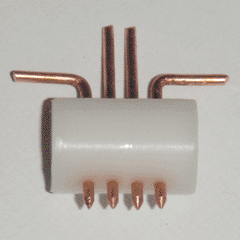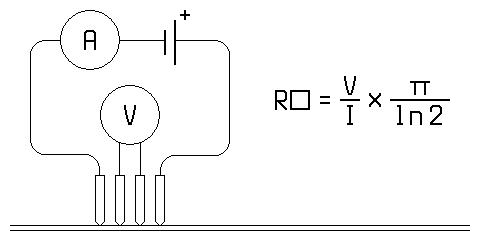Sheet Resistance ProbeOne important metric for thin films is the sheet resistance, which is often written in units of ohms per square. This corresponds to the resistance between two opposite edges of a square of the material being measured, where the square can be of any size. Fortunately, this value can be easily calculated with the use a four-point probe, as seen above. If a known current is passed through the film between the external contacts, and the voltage is measured between the internal contacts, the sheet resistance is simply [v/i * pi/ln(2)]. This assumes that the pin spacing is equal, the film thickness is much smaller than the pin spacing, and the pin spacing is much smaller than the film width. These conditions are easily achieved, however.

Construction of the probe was very straightforward; the body was made of Delrin rod and the pins were made of straightened copper wire, sharpened to a point and spaced 1/8 inch apart. The external circuit was equally simple, with the current being provided by a 1.5 volt D cell, and the measurements being taken by two multimeters. With the probe set up, I first tested the pieces of conductive glass from the previous two articles, followed by a piece of commercial ZnO:Al glass that was known to have a sheet resistance of less than 10 ohms per square. The results can be seen below.

Glass Sheet Resistance (ohms/sq.)
ZnO 208,699.4
ZnO:B 2,803.5
ZnO:Al (Commercial)  7.7

Obviously, the conductive glass I have produced so far is not yet competitive with commercial products. The main purpose of this probe was to provide a useful metric by which to judge the performance of future glass. Standardizing my test methods (and later my production methods) will make it easier to optimize variables such as solution concentration, dopant concentration, and deposition time, since I will be able to accurately measure the resulting changes in performance. My goal is to eventually produce glass with a sheet resistance of less than 1,000 ohms per square, which seems well within the realm of possibility. That said, even if this goal is achieved, I will continue optimizing the glass until I have exhausted all potential sources of improvement.

EDIT: 2/24/2017

While using this type of probe is the technically correct way to measure sheet resistance, a simpler method is to put two multimeter probes as close together as possible without touching, and read the resistance directly. I will be using this method from now on, as it requires no set-up and appears to be reliable and accurate.

Index